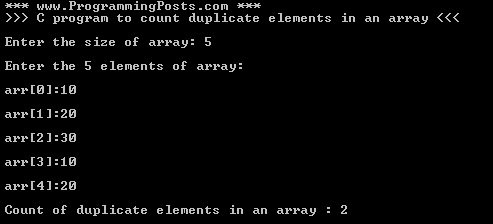# C Program to count duplicate / repeated elements in an array

### C PROGRAM TO FIND COUNT OF DUPLICATE ELEMENTS IN AN ARRAY

In this post we will see a simple C program to find the count of duplicate elements in an Array.
In below program we will get the count of elements that are duplicated i.e. elements that are occurred more than once in an array.
Example :
Suppose the elements of array are : 10, 20, 30, 10, 10
Here the element 10 is repeated 3 times in array, it means it is duplicated 2 more times and remaining elements occurred only once. Hence its output will be 2.

Program Algorithm :

Step 1: Read size of array and store it in n

Step 2: If n (i.e size of array) is greater than MAX, print message and exit program

Step 3: Read elements of array and store in arr[MAX] , here MAX is a global variable whose value is 25 in the program below

Step 4: Initialize count = 0, to avoid garbage values

Step 5: Set i = 0

Step 6: Set j = i+1

Step 7: Check for duplicate, if arr[i] = arr[j] then increment count by 1 i.e. count = count + 1

Step 8: Increment j by 1 i.e. j = j + 1 and repeat Step 7 till j<n

Step 9: Increment i by 1 i.e. i = i + 1 and repeat Step 6-7 till i<n

Step 10: Print the count and exit program

Program :

Sample output :Sample output text :

*** www.ProgrammingPosts.com ***
>>> C program to count duplicate elements in an array <<<

Enter the size of array: 5

Enter the 5 elements of array:

arr:10

arr:20

arr:30

arr:10

arr:20

Count of duplicate elements in an array : 2